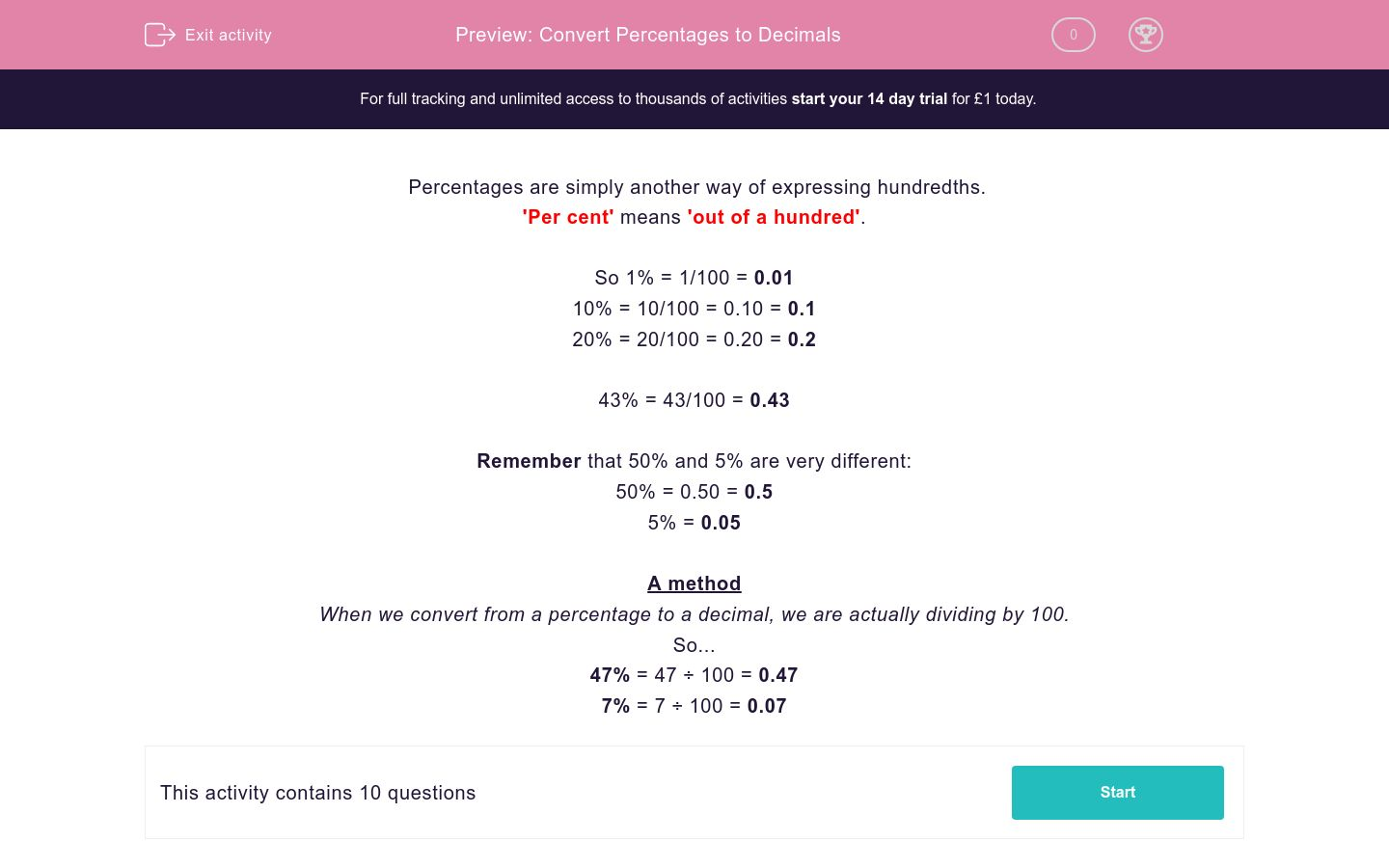# Convert Percentages to Decimals

In this worksheet, students must convert the given percentages into their decimal equivalents.Key stage:  KS 2

Curriculum topic:   Maths and Numerical Reasoning

Curriculum subtopic:   Percentages

Difficulty level:### QUESTION 1 of 10

Percentages are simply another way of expressing hundredths.

'Per cent' means 'out of a hundred'.

So 1% = 1/100 = 0.01

10% = 10/100 = 0.10 = 0.1

20% = 20/100 = 0.20 = 0.2

43% = 43/100 = 0.43

Remember that 50% and 5% are very different:

50% = 0.50 = 0.5

5% = 0.05

A method

When we convert from a percentage to a decimal, we are actually dividing by 100.

So...

47% = 47 ÷ 100 = 0.47

7% = 7 ÷ 100 = 0.07

Convert the following percentage to a decimal:

23%

Convert the following percentage to a decimal:

28%

Convert the following percentage to a decimal:

78%

Convert the following percentage to a decimal:

70%

Convert the following percentage to a decimal:

90%

Convert the following percentage to a decimal:

9%

Convert the following percentage to a decimal:

2%

Convert the following percentage to a decimal:

25%

Convert the following percentage to a decimal:

99%

Convert the following percentage to a decimal:

30%

• Question 1

Convert the following percentage to a decimal:

23%

0.23
EDDIE SAYS
23 ÷ 100 = 0.23
• Question 2

Convert the following percentage to a decimal:

28%

0.28
EDDIE SAYS
28 ÷ 100 = 0.28
• Question 3

Convert the following percentage to a decimal:

78%

0.78
EDDIE SAYS
78 ÷ 100 = 0.78
• Question 4

Convert the following percentage to a decimal:

70%

0.7
0.70
EDDIE SAYS
70 ÷ 100 = 0.70 = 0.7
• Question 5

Convert the following percentage to a decimal:

90%

0.9
0.90
EDDIE SAYS
90 ÷ 100 = 0.90 = 0.9
• Question 6

Convert the following percentage to a decimal:

9%

0.09
EDDIE SAYS
9 ÷ 100 = 0.09
• Question 7

Convert the following percentage to a decimal:

2%

0.02
EDDIE SAYS
2 ÷ 100 = 0.02
• Question 8

Convert the following percentage to a decimal:

25%

0.25
EDDIE SAYS
25 ÷ 100 = 0.25
Remember this is the same as ¼.
• Question 9

Convert the following percentage to a decimal:

99%

0.99
EDDIE SAYS
99 ÷ 100 = 0.99
• Question 10

Convert the following percentage to a decimal:

30%

0.3
0.30
EDDIE SAYS
30 ÷ 100 = 0.30 = 0.3
---- OR ----

Sign up for a £1 trial so you can track and measure your child's progress on this activity.

### What is EdPlace?

We're your National Curriculum aligned online education content provider helping each child succeed in English, maths and science from year 1 to GCSE. With an EdPlace account you’ll be able to track and measure progress, helping each child achieve their best. We build confidence and attainment by personalising each child’s learning at a level that suits them.

Get started